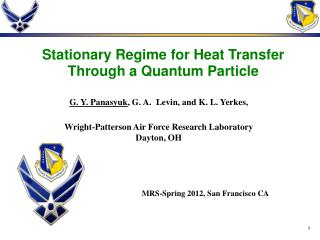DownloadDownload PresentationG. Y. Panasyuk , G. A. Levin, and K. L. Yerkes,

# G. Y. Panasyuk , G. A. Levin, and K. L. Yerkes,

Télécharger la présentation## G. Y. Panasyuk , G. A. Levin, and K. L. Yerkes,

- - - - - - - - - - - - - - - - - - - - - - - - - - - E N D - - - - - - - - - - - - - - - - - - - - - - - - - - -
##### Presentation Transcript

1. Stationary Regime for Heat Transfer Through a Quantum Particle G. Y. Panasyuk, G. A. Levin, and K. L. Yerkes, Wright-Patterson Air Force Research Laboratory Dayton, OH MRS-Spring 2012, San Francisco CA

2. Outline • HEAT CURRENT and THERMAL CONDUCTANCE between two baths through a mediating quantum system using the generalized quantum Langevin equation Both the heat baths and mediator are described in the harmonic approximation and the Drude-Ullersma model is used to describe the baths • FOURIER’S LAW for a chain of nanoparticles coupled to each other by quantum systems and HEAT FLUX between an STM tip and a substrate through a molecule

3. Heat Transport T1 > T2 T2 QS Bath-1 Bath-2 Heat flux

4. The Hamiltonian

5. Heisenberg Equations

6. Solving the Heisenberg Equations

7. Quantum Langevin equation

8. Drude-Ullersma model Is the mode spacing in a heat bath

9. Heisenberg Time Bath mode spectrum -“Heisenberg time” Stationary regime:

10. Solution of the Langevin equation Using the Laplace transform L,one finds and

11. Heat current Rate of energy change in the nth heat reservoir: where - is the work the quantum system performs on the nth bath per unit of time or the heat current

12. Final expressions for where and - the phonon occupation number for 1st and 2nd baths havingand Thermal conductance:

13. Thermal transport for different T and 1.High temperatures: 2. Low temperatures: Compare to black body radiation from area A:

14. Comparing to Stefan-Boltzmann flux one can choose In order to compare and eV CO molecule on the STM tip I. Altfeder, A.A. Voevodin, and A.K. Roy, PRL 105, 166101 (2010)

15. Fourier’s law One can envision a chain of our composite quantum systems assembled between two baths. Problem: Fourier’s law breaks if coupling is strong (Y. Dubi, M. Di Ventra, PRE, 2009) Fourier’s law can be restored if one considers a chain of large but finite subsystems (nanoparticles) coupled to each other by our quantum mediators: Fornth nanoparticle:

16. Fourier’s law (continuation) Fourier’s law is restored: If If, for example, and Again, if no ‘jumps’, Fourier’s law is restored

17. T-profiles for high and low T

18. Conclusions • We considered heat transport between two heat baths mediated by a quantum particle using the generalized Langevin equation. Both the heat baths and mediator are described in the harmonic approximation and the Drude-Ullersma model is used to describe the baths. The expressions for the heat current and thermal conductance are valid for arbitrary coupling strength between the mediator and baths and arbitrary Debye cutoff frequency. • We analyze our results for different temperature regimes and different coupling strengths. The results are also applied to a model of a chain made of macroscopically large but finite subsystems described by the Drude-Ullersma model and coupled to each other through the quantum mediators. We find that Fourier’s law is restored even for a case of strong coupling. • Heat exchange across a sub-nanometer size gap between an STM nanotip and a substrate filled by the molecule was considered providing a possible explanation of anomalously large heat flux observed in the experiment.

19. We acknowledge support from: • National Research Council • Wright-Patterson Air Force Research Laboratory, Dayton, Ohio • Thank you!